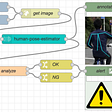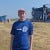# Work with integers as binary in C++

Sometimes we need to work with integers in binary format and manipulate individual bytes in their binary representation. Here I will show two ways we can work with integers this way.

First way is to use ordinary int type.

First let’s create two variable in binary format:

`int x = 0b00100;int y = 0b00101;`

Here we use use binary literals with ‘b’ character. These binary literals were standardized in C++14.

We can add two values like that

`int z = a + b;`

Result is 0x01001 or 9

If we print value with cout we will get decimal representation

`cout << z << endl;9`

To print the result in binary foramt we need to use bitset library. Import it

`#include <bitset>`

and print the variable

`cout << std::bitset<6>(z) << endl;`

Here first non-type template parameter specifies the number of bits to store. 6 in our case.

Second way is to use byte type available since C++17. Notice according to documentation that it is not a character type and is not an arithmetic type. A byte is only a collection of bits, and onliy bitwise operators defined for it. Thus arithmetic ones can’t be used for it.

`#include <cstddef>`

Create byte object using regular int

`byte myByte{ 2 };`

Apply 2-bit left shift

`myByte <<= 2;`

and print result as int:

`cout << to_integer<int>(myByte) << endl;`

We can initialize variable using binary literal

`byte byte1{ 0b0011 };byte byte2{ 0b1010 };`

Apply bitwise OR and AND operations

`byte byteOr = byte1 | byte2;byte byteAnd = byte1 & byte2;`

and print result as int:

`cout << to_integer<int>(byteOr) << endl;`

That’s all you need to work with binary numbers in C++.

--

--

--

Love podcasts or audiobooks? Learn on the go with our new app.

## “Wiki” — The First Truly Global Language?## TryHackMe — RazorBlack## Publishing Tekton Resources as bundles on OCI registry## Human pose estimation using Node-RED## GraphQL? What?## My journey from the world of Java and Python to C++## The differences between Static and Dynamic Libraries